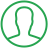# Loop With Index Python

Last Updated : Oct 6, 2023In this article we will show you the solution of loop with index python, using a for loop, lists, tuples, and other iterable objects are frequently looped over in Python. Accessing each element's index and value within the iterable could occasionally be necessary.

The index of an element in a list or tuple, which starts at 0 for the first element and increases by 1 for each additional member, allows you to retrieve that element's value in Python.

Within a loop, you can access each element's value by using this index. Now we'll talk about the looping idea in index Python.

## Step By Step Guide On Loop With Index Python :-

### Method - Accessing the values of the index elements

```data = "TALKERSCODE"
print("Index values and Indices in the List:")
for i in range(len(data)):
print(i, data[i])
```
1. Here, you can see how Python was used to write the looping code.
2. We initialise a string variable called data with the value "TALKERSCODE" at the beginning of the function.
3. The console is then written with the words "Index values & Indices in the List:".
4. The range function is then used to start a for loop using the input len(data).
5. A series of numbers between 0 and the length of the data string minus one are returned by this function.
6. Each value from the series is assigned to the variable i by the loop.
7. The expression data[i] in the loop outputs the value of i (the index value) as well as the character in the data string that is located at that index.
8. The loop then continues until all of the data string's indices have been handled.

### Method - Accessing index with the enumerate() method

```data = ["java", "python", "HTML", "PHP"]
print("List of indices and values")
for i in enumerate(data):
print(i)
```
1. You can see that we use the enumerate() method to obtain the index while writing the looping Python code in this example.
2. A list variable called data is initialised with four string values at the beginning of the code.
3. After that, the "List of indices & values" is printed to the console.
4. After that, a for loop is started using the built-in enumerate() method to run through the data list.
5. The data list is passed to the enumerate() function, which returns a sequence of tuples, each of which has two elements: the index of the current item and the actual item.
6. The print() method is used inside the loop to output the tuple for the current iteration.
7. The first member of this tuple represents the index of the current item, while the second element is the actual item.
8. The loop terminates when every item in the data list has been processed.

## Conclusion :-

Therefore, using index Python, we were able to successfully learn the concept of loop.

The fact that each element in an iterable may be accessed using a variety of methods for both index and value was another thing we learned.

One method is to access each element's value within a loop by its index.

Using the built-in enumerate() function, which returns an iterable with tuples of the index and value of each element in the original iterable, provides an alternative strategy.

Based on the particular use case, each approach has benefits and drawbacks.

When working with huge or complex iterables, using the index can sometimes be easier, but it can also be less concise and more difficult to read.

However, using enumerate() may require greater processing and memory capacity and make the code less readable and concise.

I hope this article on loop with index python helps you and the steps and method mentioned above are easy to follow and implement.

##About Amruta

Amruta is an Experienced web developer with 4 years for experience she completed her master's with MCA and passionate about programming Languages for creating technical contents like HTML, CSS, JavaScript, Java, Python, PHP, jQuery.